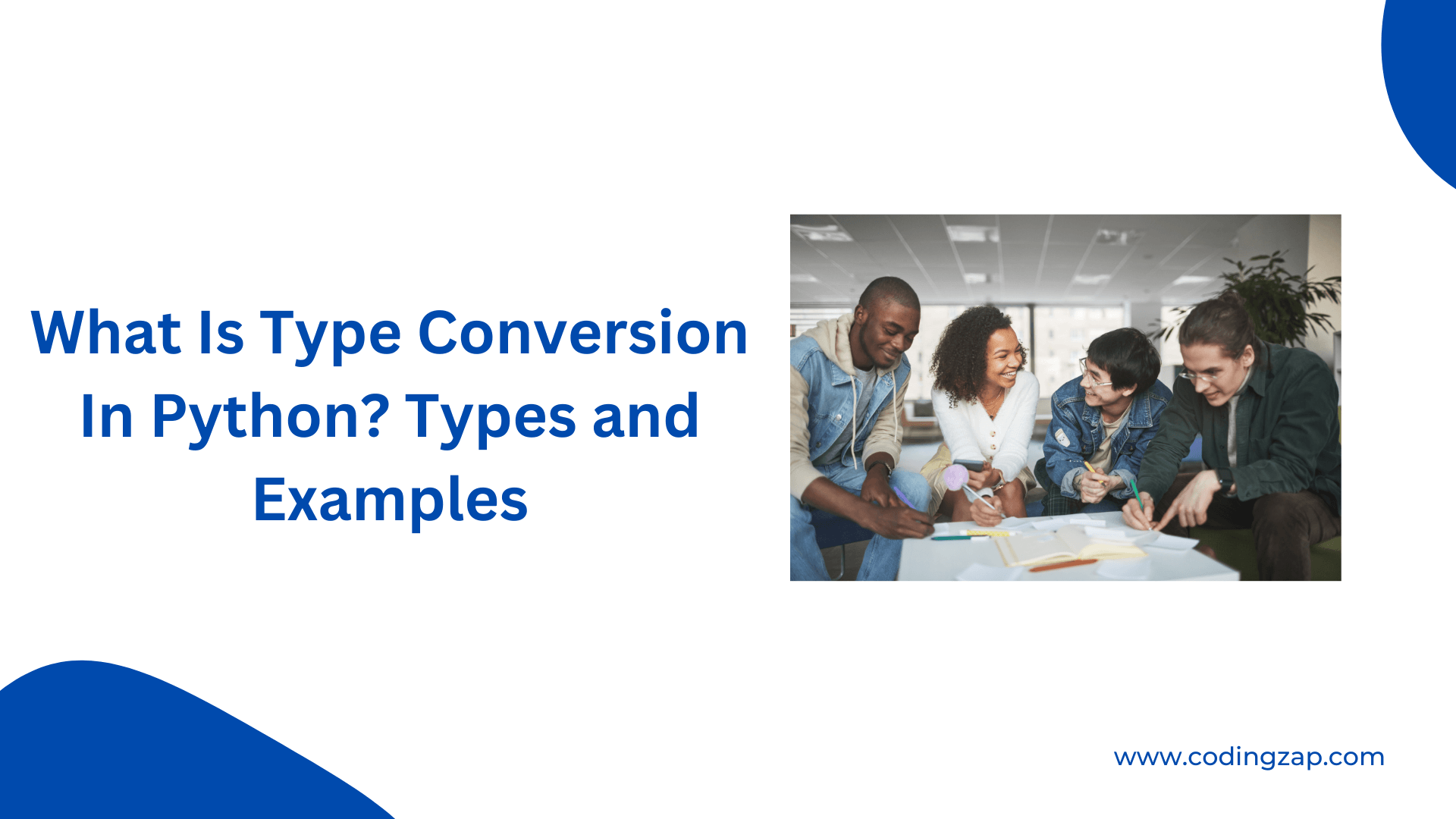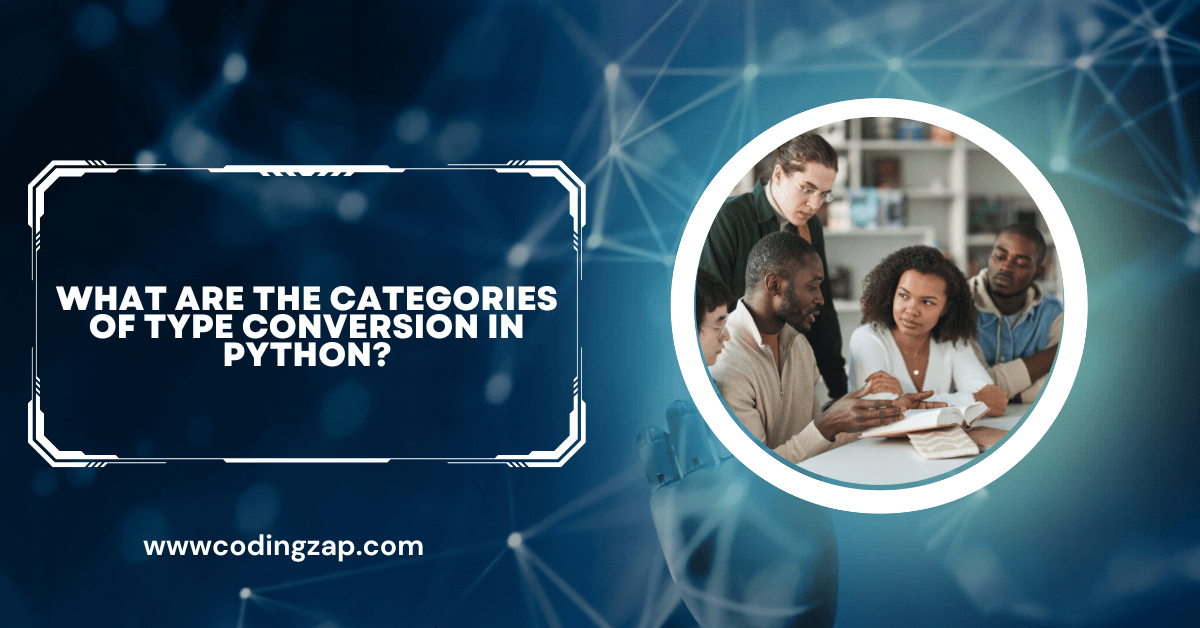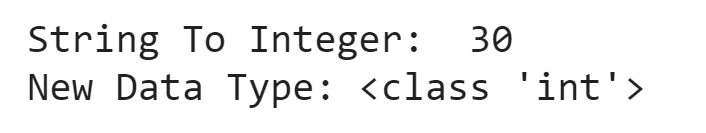# What Is Type Conversion In Python? Types and Examples Explain

Do you know what is type conversion in Python? Have you ever thought about how to do type conversion in Python? So, in this article, we will try to find out implicit type conversion & explicit type conversion in Python programming.

But before we start writing about type conversion in Python, let us first try to know what is the conversation system with the help of daily life examples. After going through this example, we will try to find out the details of implicit type conversion & explicit type conversion. Also, if you are studying Python coursework in your college and having any challenges in your homework then you can get help with your Python programming homework.

Now let’s jump to our main topic. Let us assume one scenario.

##### Real-Life Example To Explain Type Conversion In Python:

Suppose, you have visited one famous foreign country. You have booked one travel agency. And it is helping & guiding you in that foreign country. They are paying all the tickets & sightseeing costs on your behalf of you.

But as you visited one foreign country, you have decided to buy something. Now, when you went to the market for buying something, you find out that the Indian Rupee currency doesn’t work there.

So, what will you do? Do you visit the bank to exchange Dollars with a certain currency? No, you don’t need to do that. In this digital world, there is no need to worry. You have asked the seller if he accepted the Debit cards. So, with the help of an international debit card, you can pay the expenses.

There is no need to convert the currency there. The same thing happens in type conversion in Python. There is no need to typecast a data type to another data type.

We will know more about these in this topic. But let us gain knowledge of the meaning of type conversion in Python. After having that knowledge, we will find out the divisions there. Let us start from scratch.## What Is Python Programming Language? Read Below

Before we start with the type conversion in Python programming language, we have to first look at the basics of the Python programming language. Though many of you know very well about the Python programming language. But still, before moving deep into the topic, we need to understand the basic need of the Python programming language.

We all are aware of how important learning Python is, but still, if you want to know more then you can check out our article on “5 Reasons Why To Learn Python“.

There is typically no parameter present to define the Python programming language. It is neither an easy nor difficult programming language. It is considered one of the shortest programming languages. For printing any statement, there is a need to write only one line in Python programming language.

You can also check out the article ‘How To Compare Strings In Python’ to enhance your knowledge in the field of Python.

## What Is Type Conversion in Python? Get To Know

In every programming language, there are several data types are present. Like there are integers, strings, characters, etc. The programmer needs to declare the data type before declaring one variable.

As every variable of different data types is different from the other. An integer variable can’t able to go with the string variable. If one thinks to add one integer to one string, then it is not possible at all.

This is the rule for every programming language. But sometimes, there is a need to add or make collaborative operations in different data types.

So, the programmer does the typecasting process usually. But Python is a high-level programming language. This programming language is used in many corporate for developing some big projects.

So, there must be some features to remove these problems. The type of conversation in Python is the solution for that. In Python programming language, there is no need to do typecasting in every field.

Some of the common typecasting can be done by itself. This is known as type conversion in Python. There are certain types present. Now, we will look for that.

## What Are The Categories Of Type Conversion in Python?

There are mainly two categories present in type conversion in Python. In one case, there is no need to involve any individual to convert one data type to another. This means the system is capable of do changes in the program.

And in another case, programmers need to write the piece of program in such a way that, the need for typecasting completes there.So, depending on the process, we can divide the type of conversation in Python programming language into two categories. They are:

1. Implicit type conversion
2. Explicit type conversion

### Implicit Type Conversion in Python Programming Language:

This is the type of conversion method, where there is no need to declare any process in the program. The program is capable to find out the need to do typecasting there. This means, here the program finds out the line where a typecasting might be needed.

In the program, the conflict area is first identified by the compiler. And if the conflict can be resolved by the interpretation of the compiler, then the type conversation in Python programming language will be the implicit type conversion in Python programming language. But there is a certain limitation in the process.

The implicit type conversion in Python programming language can only be done in some low-category data types. Or the implicit type conversion in Python programming language can be implemented to those data types that resemble similar ones.

In the implicit type conversion in Python programming language, the two data types will not be completely different from each other. You will get more about this process when we discuss the implementation process of implicit type conversion in Python programming language.

##### Example:

In this case, we have first declared two types of data types. Here one is the integer data type & another is the float data type. These are the data types that are closely related. One data type handles the integer form of the number. And another one deals with the decimal number. Moreover, they are dealing with numbers.

So, they are the same in this context. Now, we need to do type conversion in Python programming language to do some more operations.

After declaring the variables, we are just adding those details without doing any changes to the data type. We are going to the straightforward format. We are not doing any changes there. As this type of conversion in Python programming language falls under the implicit format. It will do the necessary changes by itself.

And we will find a result without error. And the result data type will be in float. So, there was no error while doing this operation.

Here, one thing we should notice is that the output is coming in the form of a flat format. It is not coming in the integer format. Because, if the float number is converted to the integer format, then there will be a chance of loss of the data.

But if any integer is changed to the float format, then there will not be any chance of losing any data. So, from this perspective, the compiler is automatically changing the data type to the necessary one. In a certain format, where there will be no data loss.

```coding = 10 # Declaration Of Integer

zap = 10.33 # Declaration Of Float

one = coding + zap

print("Value:",one) # New Value And Data Type

print("Data Type:",type(one))```

Let us try to find out the output of the above code. This will help to find out the process of implicit type conversion in Python.

##### Output:### Explicit Type Conversion in Python Programming Language:

Now, it is time to discuss another method of type conversion in Python programming language. This is known as the Explicit type conversion in Python programming language. The Explicit type conversion in Python programming language is not similar to the previous one. Here, all tasks need to be executed by the programmer itself. The compiler is not able to do the necessary changes on its own. Because the changes are not possible to identify by the compiler. We will know its reason when we discuss more explicit type conversion in Python programming language.

In explicit type conversion in Python programming language, two data types can be changed forcefully. Those two data types might not be close to each other. Might be one data type representing one integer number. And another data type is representing one string value. So, those are not close data types. But those data types can be typecasted using the Explicit type conversion method in Python programming language. For that reason, some data might be removed from the existing data. We will know more about those when we implement some of them.

There are certain methods present to implement the Explicit conversion method in Python programming language. Each & every one of them can be used for certain pairs of data types. We will also mark those data types in which we can use those functions or methods. Let us make a list of the functions that are present in the Python programming language as an inbuilt function.

• Int() Function: This is the inbuilt function that is used to convert any data type to the integer function. It is only the function that can be utilized for any data type conversion in Python programming language.
• Float() Function: It is another data type conversion function in Python programming language. As the name suggests the function is needed to convert any data type to a float number. This function also can be used for any data type.
• Str() Function: This is another function that is used for the conversion of integer data type to the string data type in Python programming language.
• Ord() Function: This is the function that is used for a character to integer type conversion in Python programming language.
• Chr() Function: This is the function that is used for integer to character type conversion in Python programming language.
• Hex() Function: As the name suggests the inbuilt function is implemented to do integer to hexadecimal string type conversion in Python programming language.
• Tuple() Function: This is another function that is used for the conversion of any data type to the Tuple structure in Python programming language.
• Oct() Function: This is the inbuilt function that needs to be used to do integer to octal string type conversion in Python programming language.
• Set() Function: This is another function that is used for the conversion of any data type to the Set structure in Python programming language.
• List() Function: This is another function that is used for the conversion of any data type to the List structure in Python programming language.
• Dist() Function: This is another function that is used for the conversion of Tuple data to the Dictionary structure in Python programming language.
##### Example 1: Int() Function Implementation:

It is time to convert any data type to the integer format. For that, we have declared a string & an integer variable. Now, we need to add those variables to each other. This can’t be possible like the above process. Here, we need to convert the string to an integer, and then the addition of those two variables is possible. And the result will be of integer type.

General Syntax: int(string-variable)

```zapone = '10' # Declaration Of String

codingzap= 20 # Declaration Of Integer

one = int(zapone) # Explicit Conversation

print("String To Integer: ",one + codingzap)  # New Value And Data Type

print("New Data Type:",type(one))```

Let us try to find out the output of the above code. This will help to find out the process of explicit type conversion in Python.

##### Output:##### Example 2: Ord() Function Implementation:

Now, we will convert one character to the integer format. In this case, the ASCII value of the character will be printed as the integer. So, we have declared one character. After that, we used the Ord() function & share the name of the character as the argument. Now, the result is stored in one variable. It is time to print the variable & get the result.

General Syntax: ord(character-variable)

```CodingZap = 'A' # Declaration Of Character

ZapOne = ord(CodingZap) # Character To Integer Conversation

print ("Character To Integer Conversion : ",ZapOne) # Printing The ASCII Value```

Let us try to find out the output of the above code. This will help to understand the concept more easily.

##### Output:##### Example 3: List() Function Implementation:

Now, we will convert one string to the list format. The list is the special data structure or the format that is present in the Python programming language. Here, we have declared one string. And without hesitating, we will use the list function there. For that, we need to share the name of the string as the argument to the list function. Now, the result is stored in one variable. It is time to print the variable & get the result.

General Syntax: list(string-variable)

```string = 'ZapOne' # Declaration Of String

CodingZap = list(string) # String To List Conversation

print ("String To List Conversion : ", CodingZap) # Printing The List

```

Let us try to find out the output of the above code. This will help to understand the concept more easily.

##### Output:## Conclusion:

As we saw, it is very important to know the type conversion in Python programming language.

We have to remember the divisions of the conversation policy. As well as we need to practice these things as much as possible.

It is advisable to clear the basic concept of the Python programming language. Then it will be easy for us to understand the topic. This will help us in the future.

So, hope you have liked this piece of article. Share your thoughts in the comments section and let us know if we can improve more.

If you are curious to learn other topics on Python then we have written some useful and detailed articles on the below topics: